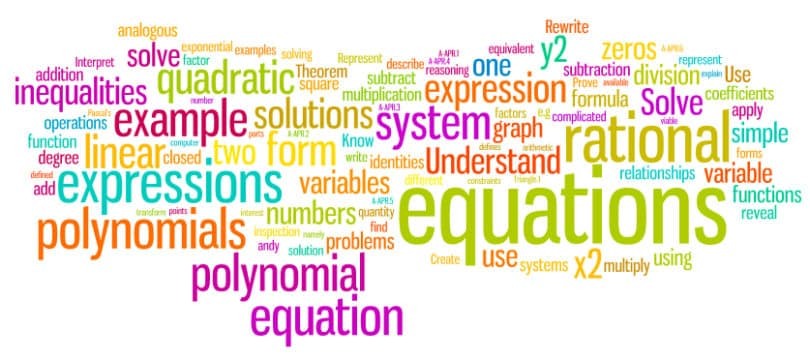Engineering Entrance Sample Papers

BSAUEEE Maths Questions for PracticeThis Practice sample paper is based on BSAUEEE syllabus and consist 34 questions, you can download all 34 questions in PDF format using link below last question.

Ques. Let PQR be a right angled isosceles triangle, right angled at P(2, 1). If the equation of the line QR is 2x + y = 3, then the equation representing the pair of lines PQ and PR is
(a) 3x2 – 3y2 + 8xy + 20x + 10y + 25 = 0
(b) 3x2 – 3y2 + 8xy – 20x – 10y + 25 = 0
(c) 3x2 – 3y2 + 8xy + 10x + 15y + 20 = 0
(d) 3x2 – 3y2 – 8xy – 10x – 15y – 20 = 0
Ans:- (b)

Ques. Let R = {(3, 3), (6, 6), (9, 9), (12, 12), (6, 12), (3, 9), (3, 12), (3, 6)} be a relation on the set A = {3, 6, 9, 12}. The relation is
(a) An equivalence relation
(b) Reflexive and symmetric only
(c) Reflexive and transitive only
(d) Reflexive only
Ans:- (c)

Ques. Which of the following pieces of data does not uniquely determine an acute angled △ABC (R = circum-radius)
(a) a, sin A, sin B
(b) a, b, c
(c) a, sin B, R
(d) a, sin A, R
Ans:- (d)

Ques. How many words can be made from the letters of the word INSURANCE, if all vowels come together?
(a) 18270
(b) 17280
(c) 12780
(d) None of these
Ans:- (d)

Ques. Let function f (x) = x2 + x + sin x – cos x + log (1 + |x|) be defined over the interval [0, 1]. The odd extensions of f(x) to interval [­–1, 1] is
(a) x2 + x + sin x + cos x – log (1 + |x|)
(b) –x2 + x + sin x + cos x – log (1 + |x|)
(c) –x2 + x + sin x – cos x + log (1 + |x|)
(d) None of these
Ans:- (b)

Ques. If the coefficient of the second, third and fourth terms in the expansion of (1 + x)n are in A.P., then n is equal to
(a) 7
(b) 2
(c) 6
(d) None of these
Ans:- (a)

Ques. The binary equivalent of octal number (5473.64)8 is
(a) (110100111011.110101)2
(b) (101100111011.110100)2
(c) (100100111011.110100)2
(d) (101100111101.110100)2
Ans:- (b)

Related: WBJEE Physics Practice Questions

Ques. If value of a third order determinant is 11, then the value of the square of the determinant formed by the cofactors will be
(a) 11
(b) 121
(c) 1331
(d) 14641
Ans:- (d)

Ques. A horizontal rod AB is suspended at its ends by two vertical strings. The rod is of length 0.6m and weighs 3 Newton. Its centre of gravity G is at a distance 0.4m from A. Then the tension of the string at A is
(a) 0.2 N
(b) 1.4 N
(c) 0.8 N
(d)  1 N
Ans:- (d)

Ques. If the range of any projectile is the distance equal to the height  from which a particle attains the velocity equal to the velocity of projection, then the angle of projection will be
(a) 60 degree
(b) 75 degree
(c) 36 degree
(d) 30 degree
Ans:- (b)

Ques. If the coefficient of correlation is positive, then the regression coefficients
(a) Both are positive
(b) Both are negative
(c) One is positive and another is negative
(d) None of these
Ans:- (a)

Ques. If the binary operation * is defined on a set of ordered pairs of real numbers as (a, b) * (c, d) = (ad + bc, bd) and is associative, then (1, 2) * (3, 5) * (3, 4) =
(a) (74, 40)
(b) (32, 40)
(c) (23, 11)
(d) (7, 11)
Ans:- (a)